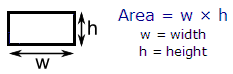# Calculate Square Feet and inches - use this online calculator

Below you can use an online calculator to help you calculate square feet and inches. You have to enter the height and length of a square or rectangle. You can include feet and inches. The calculator will give you the resulting square footage and equivalent square inches.You may enter only feet, only inches or a combination of both in either the width or length fields. The total width and length will be updated when you click the 'Calculate' button.  You may also wish to change just certain fields and recalculate.

### How to Calculate Square Feet and inches

Example

Height =     10 ft  4 in
Length =     9  ft  3 in

The results are:

Heigth (ft) =  10.333
Length (ft) =   9.250
Square Feet =  95.583 ft2

Heigth (in) =       124
Length (in) =       111
Square inches =  13,764  in2

 Please, enable JavaScript code, otherwise the calculator won't work.

 Enter height and length of your area... Height = ft in Length = ft in Height (ft) = Length (ft) = Area = ft2 Height (in) = Length (in) = Area = in2

From 'Calculate Square Feet' to Matlab home

From 'Calculate Square Feet' to Free Online Calculators